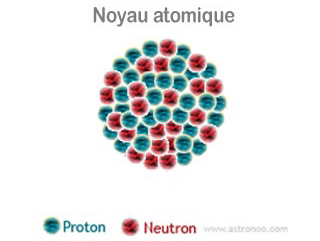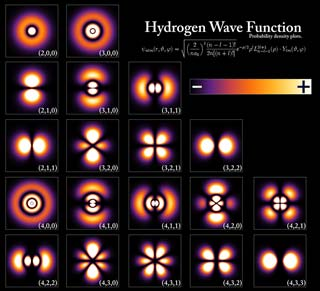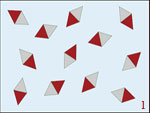# Atom

## Structure of the atomAutomatic translation Category: matter and particles
Updated November 06, 2013

Everything we see is made up of atoms, many atoms. It was watching the smallest constituents of the matter that scientists have been able to explain, in the twentieth century, the operation of the entire universe. An atom is constituted by a core around which moves one or more electrons. What characterizes the core is its number of protons ( Z) ranging from 1 to 110, it is it who determines the element, such as iron (FE26) has 26 protons, 26 is the atomic number. The number of neutrons (N) ranging from 0 to 160, characteristic of the isotopes of the element, for example, hydrogen (H1) has a proton and no neutron, deuterium (H2) has a proton and a neutron, tritium (H3) has a proton and two neutrons. These three forms of hydrogen have only one electron, since there is only one electrical load, the single proton. Attention that it is only in the case of hydrogen that is given a different name to the isotopes of the element, in all other cases we indicate only the number of nucleons thereby find the number of neutrons. For example iron (FE26) has several isotopes Fe56, we understand that Fe56 has 30 neutrons, Fe57 has 31 neutrons, Fe58 32 neutrons, the number of neutrons differs well isotopes.
In the atom are electrons that give consistency to the material, yet it is very light, its mass is about 10-27 grams, the proton is 2000 times heavier and concentrates majority of the mass of the atom (99.99 % ). For stable atoms, the mass is between 1.674 × 10-24 g for Hydrogen and 3.953 × 10-22 g for uranium. Since 1811, we also know the approximate size of an atom, Amedeo Avogadro (1776-1856) estimated the size of atoms at 10-10 meter, i.e. a little more than 10 millionths of a millimeter.

In 1911, Ernest Rutherford (1871-1937) discovered the atomic nucleus and specifies the structure of the atom by bombarding gold foil with particles from the radioactive decay of uranium. He gives a nucleus size in the order of 10-14 meters. There is a little more than one hundred different atoms, these are the elements such as hydrogen, carbon, oxygen or iron. The New Zealand physicist had the idea of a representation of the atomic nucleus. Rutherford represents each atom as a mini solar system, the center and the nucleus like planets orbit the electrons. The nucleus itself is represented as a mature grains (picture to the right). This pictorial representation is false but has two advantages, it clearly differentiates the two particles, the proton and neutron and we understand that  the core, very compact, is circumscribed within a defined volume. But since the advent of quantum mechanics in the 1920s, the nucleus image is disturbing, the nucleus is no longer a system of balls associated together. The nucleus is governed by quantum mechanics, in other words it exist than if it is observable but observe the protons and neutrons inside the nucleus as they are in the picture, is not possible because it would illuminate the particles with a light so intense that the nucleus would instantly disintegrate. This representation in form of grains blackberry hides the quantum concept of matter. It is the same for the electron, no longer represents the electron as a particle which rotates on a very regular orbit around the nucleus. The electron is both a wave and a particle, the wave-particle duality is the foundation of quantum mechanics. In quantum mechanics the electron does not follow a single path, it is located in a region around the nucleus, called the electronic cloud or atomic orbital.## Electron cloud or atomic orbital

Since 1924, all matter is associated with a wave, is the assumption of Louis de Broglie (1892-1987). With this hypothese, he generalizes to all the particles of matter, wave-particle duality brought to light by Max Planck (1858-1947) in the early 20th century. All subatomic particles therefore have a wavelength.
The wavelength λ of a subatomic particle and momentum p are related by the equation: λ = h / p, where h is Planck's constant, the momentum p i.e. product of the mass by the speed vector (p = mv). Since we known, thanks to Einstein's famous formula that all matter has an associated energy (E = mc2). In other words, more the wavelength is small and more energy is high (E = h / λ). This energy will modify the shape of atoms. The foundations of quantum mechanics are then posed.
In short, matter is really composed of very small particles, fermions (electrons, neutrinos, quarks) that have mass, charge, energy, dimension, a wave, a spin. But what these particles resemble, in the world of the infinitely small ?
In 2013 we still can not see the particles of the atomic nucleus but only the outer layer of the atom i.e. the electron cloud. The electron cloud occupies all the spatial extent of the atom as it is about 10 000 times greater than its nucleus. In wave quantum mechanics, a particle is represented by a wave function, but it is very difficult to represent the fundamental concept of quantum mechanics or quantum state of a system. In 1927, Max Born (1882-1970) gave an interpretation of the wave function where the square of the wave function represents the probability, when you make a measurement, finding the particle at a specific location function.
A wave function is an amplitude or a probability density function of the presence system in a given position at a given instant. This function has a complex value. If a real number or an actual value is, for example, the length of a segment on a straight line, a complex value is represented by a vector in a plane, this vector has not only a length in space but also a phase which corresponds to the direction of the vector.

If you no longer represents the electron as a point particle on a regular orbit around a nucleus, how can you make a picture?
Well here, the electron does not follow a single path around the nucleus, it is somewhere in a vast region that we call the electron cloud or atomic orbital. The state of an electron is represented by the volume of the space around the nucleus in which it is localized. The fundamental state of hydrogen is about one angstrom is 10-10 meters. To represent the electron in this region just imagine a grain of rice of about 5 mm to move in a sphere about 50 meters in diameter. In addition, the shape of this region of atomic space depends on the energy of the electron and its momentum is what we see on the image cons. Thus the orbital of the electron characteristics may take various forms depending on the nature of the atom, for example, the orbital of the hydrogen atom on the first row at the top has a spherical shape, the orbital second row has the shape of two drops of water, the third row on the orbital in the form of four drops of water. In summary, in the orbiting region of the space where the electron is delocalized, the electron state is a superposition of all possible positions within the atomic orbital whose shape varies. The shape of the orbital changes when excited atom, as in the first row. If excites even more atom, the shape of the orbital change again as the second row or electronic layer. In a very excited state called "Rydberg state", electrons are delocalized in a torus "large radius" that can measure up to 1000 angstroms, the principal quantum number n (number of layer) is very high between 50 and 100.

nota: an electron attracted by the positive charge of the nucleus, can not "stick to the nucleus" because it would mean that the spatial extension of the wave function is reduced to a point. The Schrödinger equation says that an electron in the vicinity of the nucleus is in a orbital to geometry determined by the quantum numbers which satisfy this equation. In short, an electron is confined in the vicinity of the nucleus by the electrostatic potential well. When the potential energy is increasing, we say that the particle moves in a potential well.### Image: Representation of the first electronic orbitals of hydrogen based on the energy of the electron and its angular momentum, the energy level increases from top to bottom (n = 1 , 2, 3) and the momentum increases from left to right , (I = s , p, d , f , g) . This image shows the probability density of finding the electron, the black color represents the density 0, i.e. the area where the electron will never adventure. The white color represents the maximum density, i.e. the area where the electron passes most often. Between black and white in the orange-red zone, the probability density increases. Quantum numbers are represented by letters n, is the principal quantum number, it defines the energy level of the electron. I is the orbital quantum number, or secondary, as it defines the electronic sublayers, s (sharp) for l = 0 , p (principal) for l = 1 , d (diffuse) for l = 2 , f (Fundamental) for I = 3 , then (to the excited states ) g , h , i , ... the quantum number m is the magnetic quantum number or tertiary .Credit image: GNU Free Documentation License.

 1997 © Astronoo.com − Astronomy, Astrophysics, Evolution and Earth science. Contact    Mentions légalesScale of the nanoparticles...Neutrino
and beta emission...Magnetic order
and magnetization...Leap second...Windows
of the spectrum...